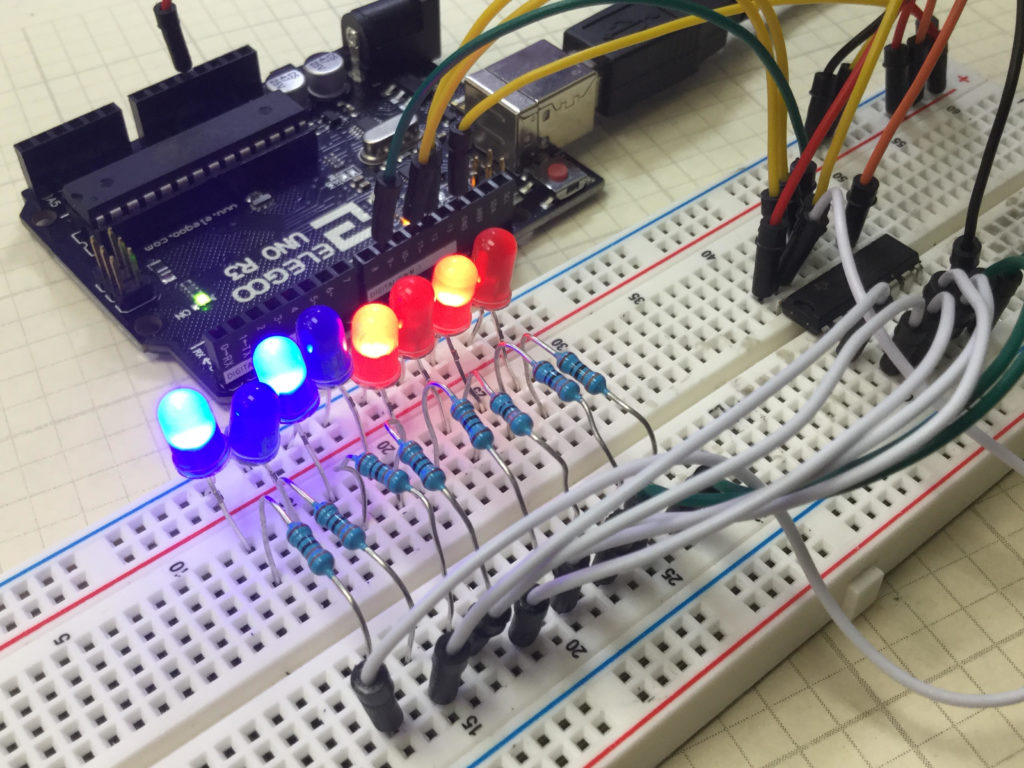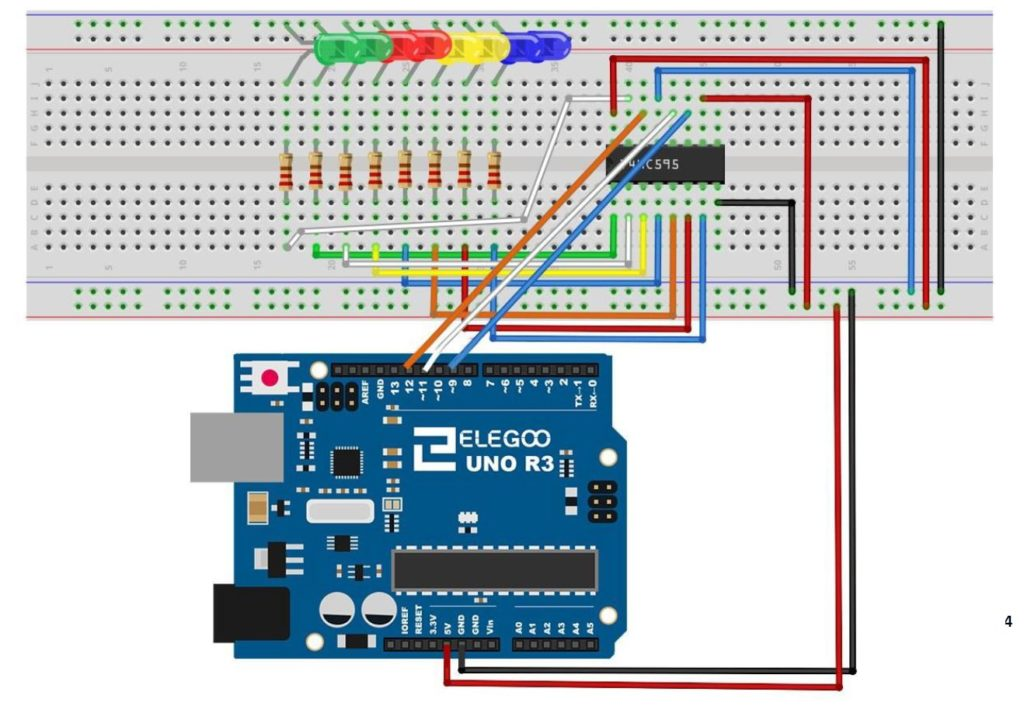# Arduino Tutorial 43: Binary Counter with 74HC595 Serial to Parallel Shift Register

In lesson 42 we showed you how to connect and program the 74HC595 shift register. We showed how data in byte format would then be written to an array of 8 LED to give a visual representation of the binary version of that byte variable. We then gave you the assignment to create a Binary Counter using the 4HC595. In this lesson we show you the solution. This builds on Lesson 42, so make sure to have your basic 74HC595 circuit set up before starting this lesson.

In this lesson we are using parts from the Elegoo Arduino kit, which you can get  HERE.

The code we ended up developing in this lesson is provided below.

# Arduino Tutorial 42: Understanding How to Use a Serial to Parallel Shift Register (74HC595)In this Circuit the 74HC595 is independently controlling 8 different LEDs.

In this lesson we show you how to expand the number of LEDs or other devices you can control with the Arduino by incorporating a Serial to Parallel converter. The chip we will be using is the 74HCH595. When connected to just a few pins of the Arduino, data can be sent serially to the chip, and then LEDs can be connected to the output pins of that chip. Hence, you can control 8 LEDs using only 3 digital pins on the Arduino.

This is somewhat of a tedious project, because the circuit has lots of wires, and it must be connected perfectly. We use the following schematic in this project:This is the schematic we use in this example to control 8 LEDs from the 74HC595 chip.

The video takes you step by step through the entire build and programming.

The code we used in this build is included below: